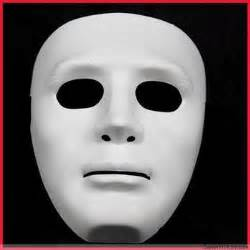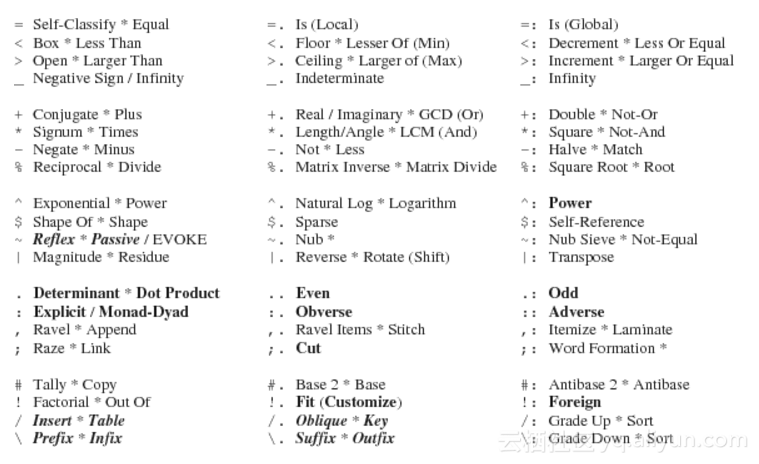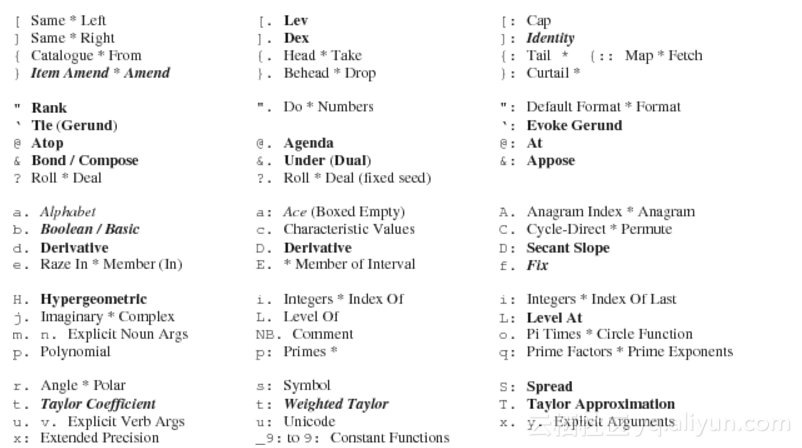# 函数式编程和 J 编程语言 【已翻译100%】（2/3）青衫无名 2017-06-02

3 为什么要学习函数式编程

3.1 可任意赋值

3.2 抽象层次高

3.3 并行计算

3.4 人工智能

3.5 原型设计

3.6 理论

4 J函数式编程语言

J编程语言[Burk 2001,Bur 2001,Hui 2001]是一个函数式编程语言。J使用插入记号和特殊符号表示原函数，诸如+或者 %，或者在 .或者 :后面添加特殊符号或者单词。每个函数名称可能作为一元参数（一个变元，通常写在右边）或者二元参数（两个变元，一个在左边，另一个在右边）。

J语言内置函数列表在数字1和2里展示。这些数字展示了在*左边的一元函数定义和在右边的二元函数定义。例如，函数标记+:代表一元doubl参数e和二元not-or(nor)参数。

Figure 1: J Vocabulary, Part 1J使用简单的规则判定函数表达式的优先级。一元变量或者右边的二元变量是整个表达式右边的值。左边二元变量的值作为优先项赋值给左边的二元变量。括号用于更改表达式的优先级。例如，表达式34+5值为27，然而表达式（34）+5的值为17。

Figure 2: J Vocabulary, Part 2J是一种函数式编程语言，它使用函数化组成方式模拟计算处理。J提供一种叫做隐式（tacit）的编程方式。隐式（Tacit）编程无需引用他们的参数，并且常常使用特别的构成规则，这被叫做hooksand forks。显式编程这种传统的控制结构也被支持。内部一个显式的定义，左边的参数是一对的，总是被命名为 x. 同时单参数（与右边的双参数类似）会被命名为 y. 。

J提供了一个强有力的简单数据结构集合比如（列表）list和（数组）array。数据（在J语言中撤销是函数的第一类状态），一旦将标识创建为常量或者函数应用，就不可以再修改。数据项具备这样几个属性，例如类型（type）（数字或者字符，确切值或者不确定值等等），图形（shape）（每个轴线上都有长度的列表（list））和排（rank）（轴线上的数字）。名称（Name）是一个抽象的工具（非内存单元），它可以被赋值（或者反向赋值）为数据或者函数。

5 J 语言编程示例

``````x =: 'x'
y =: 'y'
``````

``````f =: 3 : 0
'f(',y.,')'
:
'f(',x.,',',y.,')'
)
``````

``````math_pat =: 3 : 0
'''',y.,'('',y.,'')''',LF,':',LF,'''',y.,'('',x.,'','',y.,'')'''
)
``````

``````math_pat 'f'
'f(',y.,')'
:
'f(',x.,',',y.,')'
``````

``````f =: (3 : (math_pat 'f')) :. (3 : (math_pat 'f_inv'))
g =: (3 : (math_pat 'g')) :. (3 : (math_pat 'g_inv'))
h =: (3 : (math_pat 'h')) :. (3 : (math_pat 'h_inv'))
i =: (3 : (math_pat 'i')) :. (3 : (math_pat 'i_inv'))
``````

``````f g y
f(g(y))
(f g) y
f(y,g(y))
x f g y
f(x,g(y))
x (f g) y
f(x,g(y))
f g h y
f(g(h(y)))
(f g h) y
g(f(y),h(y))
x f g h y
f(x,g(h(y)))
x (f g h) y
g(f(x,y),h(x,y))
f g h i y
f(g(h(i(y))))
(f g h i) y
f(y,h(g(y),i(y)))
x f g h i y
f(x,g(h(i(y))))
x (f g h i) y
f(x,h(g(y),i(y)))
f@g y
f(g(y))
x f@g y
f(g(x,y))
f&g y
f(g(y))
x f&g y
f(g(x),g(y))
f&.g y
g_inv(f(g(y)))
(h &. (f&g))y
g_inv(f_inv(h(f(g(y)))))
x f&.g y
g_inv(f(g(x),g(y)))
f&:g y
f(g(y))
x f&:g y
f(g(x),g(y))
(f&g) 'ab'
f(g(ab))
(f&(g"0)) 'ab'
f(g(a))
f(g(b))
(f&:(g"0)) 'ab'
f(
g(a)
g(b)
))))
f^:3 y
f(f(f(y)))
f^:_2 y
f_inv(f_inv(y))
f^:0 y
y
f 'abcd'
f(abcd)
f/ 2 3\$'abcdef'
f(abc,def)
(f/"0) 2 3\$'abcdef'
abc
def
(f/"1) 2 3\$'abcdef'
f(a,f(b,c))
f(d,f(e,f))
(f/"2) 2 3\$'abcdef'
f(abc,def)
'abc' f/ 'de'
f(abc,de)
'abc' (f"0)/ 'de'
f(a,d)
f(a,e)

f(b,d)
f(b,e)

f(c,d)
f(c,e)
'abc' (f"1)/ 'de'
f(abc,de)
``````

5.1 数字

``````x: 3e10
30000000000

]a =: 1r2
1r2
(%a)+a^2
9r4
%2
0.5
% x: 2
1r2
``````

``````] matrix =: x: 3 3 \$ _1 2 5 _8 0 1 _4 3 3
_1 2 5
_8 0 1
_4 3 3

]inv_matrix =: %. matrix
3r77  _9r77  _2r77
_20r77 _17r77  39r77
24r77   5r77 _16r77

matrix +/ . * inv_matrix
1 0 0
0 1 0
0 0 1
``````

``````! x: i. 20
1 1 2 6 24 120 720 5040 40320 362880 3628800 39916800 479001600 6227020800
87178291200 1307674368000 20922789888000 355687428096000 6402373705728000
121645100408832000

! 100x
93326215443944152681699238856266700490715968264381621468592963895217
59999322991560894146397615651828625369792082722375825118521091686400
0000000000000000000000
``````

``````+/ , 5 = q: >: i. 4
0
!6
720
+/ , 5 = q: >: i. 6
1
!20x
2432902008176640000
+/ , 5 = q: >: i. 20
4
``````

J支持复数，使用符号j来区分实部和虚部。

``````0j1 * 0j1
_1
+. 0j1 * 0j1 NB. real and imaginary parts
_1 0
+ 3j4 NB. conjugate
3j_4
``````

``````1p1 NB. pi
3.14159
2p3 NB. 2*pi^3
62.0126
1x1 NB. e
2.71828
x: 1x1 NB. e as a rational (inexact)
6157974361033r2265392166685
x: 1x_1 NB. reciprocal of e as a rational (inexact)
659546860739r1792834246565
2b101 NB. base 2 representation
5
0j1
*. 0j1 NB. magnitude and angle
1 1.5708
180p_1 * 1{ *. 3ad30 * 4ad15 NB. angle (in degrees) of product
45
``````

``````rotate =: 1ad1 & *
rad2deg =: (180p_1 & *) @ (1 & {) @ *.
``````

``````rad2deg (rotate^:3) 0j1 NB. angle of 0j1 after 3 degrees rotation
93
+. (rotate^:3) 0j1 NB. (x,y) coordinates on the unit circle
_0.052336 0.99863
+/ *: +. (rotate^:3) 0j1 NB. distance from origin
1
+. rotate ^: (i. 10) 1j0 NB. points on the unit circle
1         0
0.999848 0.0174524
0.999391 0.0348995
0.99863  0.052336
0.997564 0.0697565
0.996195 0.0871557
0.994522  0.104528
0.992546  0.121869
0.990268  0.139173
0.987688  0.156434
``````

``````'x y'=: |:  +. rotate ^: (i. 360) 1j0
plot x;y
Figure 3:  单位圆的绘制

\includegraphics[width=2.85417in]{circle-plot.eps}
``````

### 5.2 算法和流程

Howland [How 1998]经常使用递归的Fibonacci函数描述递归和迭代过程。在J语言中，递归Fibonacci 定义如下：

``````fibonacci =. monad define
if. y. < 2
do. y.
else. (fibonacci y. - 1) + fibonacci y. - 2
end.
)
``````

fibonacci "0 i.11
0 1 1 2 3 5 8 13 21 34 55
Howland [ How 1998 ]也阐述了接续（即优先次序）的概念；在表达式中，一元表达式表示计算子表达式的结果

``````Given a compound expression  e and a sub-expression  f of  e, the  continuation of  f in  e is the computation in  e, written as a monad, which remains to be done after first evaluating  f.  When the continuation of  f in  e is applied to the result of evaluating  f, the result is the same as evaluating the expression  e.  Let  c be the continuation of  f in  e.  The expression  e may then be written as  c f.
Continuations provide a ``factorization'' of expressions into two parts;  f which is evaluated first and  c which is later applied to the result of  f.  Continuations are helpful in the analysis of algorithms.
``````

``````ib_work =. monad define
if. y. < 2
do. 1
else. 1 + (fib_work y. - 1) + fib_work y. - 2
end.
)
``````

``````fib_work "0 i.11
1 1 3 5 9 15 25 41 67 109 177
``````

20137人浏览
2020-07-13

0
0
1101